# Implement the classical procedure, and apply it to equation, Solve it with step sizes bf h...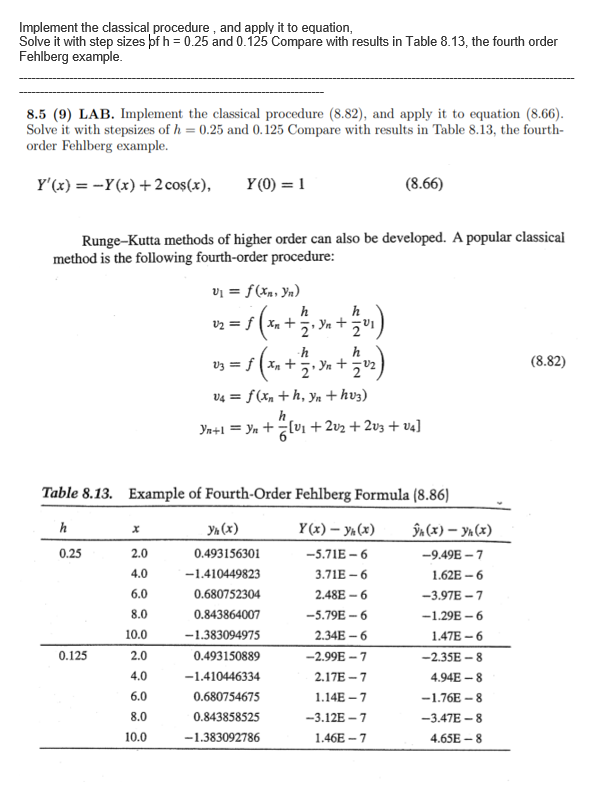Implement the classical procedure, and apply it to equation, Solve it with step sizes bf h = 0.25 and 0.125 Compare with results in Table 8.13, the fourth order Fehlberg example. 8.5 (9) LAB. Implement the classical procedure (8.82), and apply it to equation (8.66). Solve it with stepsizes of h = 0.25 and 0.125 Compare with results in Table 8.13, the fourth- order Fehlberg example. Y'(x) = -Y(x) + 2 cos(x), Y(0) = 1 (8.66) h Runge-Kutta methods of higher order can also be developed. A popular classical method is the following fourth-order procedure: Vi = f (xr, yn) h v2 = f (x+ Yn + 03 = (x + 7. Ya+202) (8.82) 14 = f (x +h, yn + huz) h Yn+1 = y + z lui + 2v2 + 2v3 + va] x Table 8.13. Example of Fourth-Order Fehlberg Formula (8.86) h yn(x) Y(x) – yn(x) (x) - ynx) 0.25 2.0 0.493156301 -5.71E-6 -9.49E-7 4.0 -1.410449823 3.71E-6 1.62E-6 6.0 0.680752304 2.48E-6 -3.97E-7 8.0 0.843864007 -5.79E-6 -1.29E-6 10.0 -1.383094975 2.34E-6 1.47E-6 0.125 2.0 0.493150889 -2.99E-7 -2.35E-8 4.0 -1.410446334 2.17E-7 4.94E-8 6.0 0.680754675 1.14E-7 -1.76E-8 8.0 0.843858525 -3.12E-7 -3.47E-8 10.0 -1.383092786 1.46E-7 4.65E-8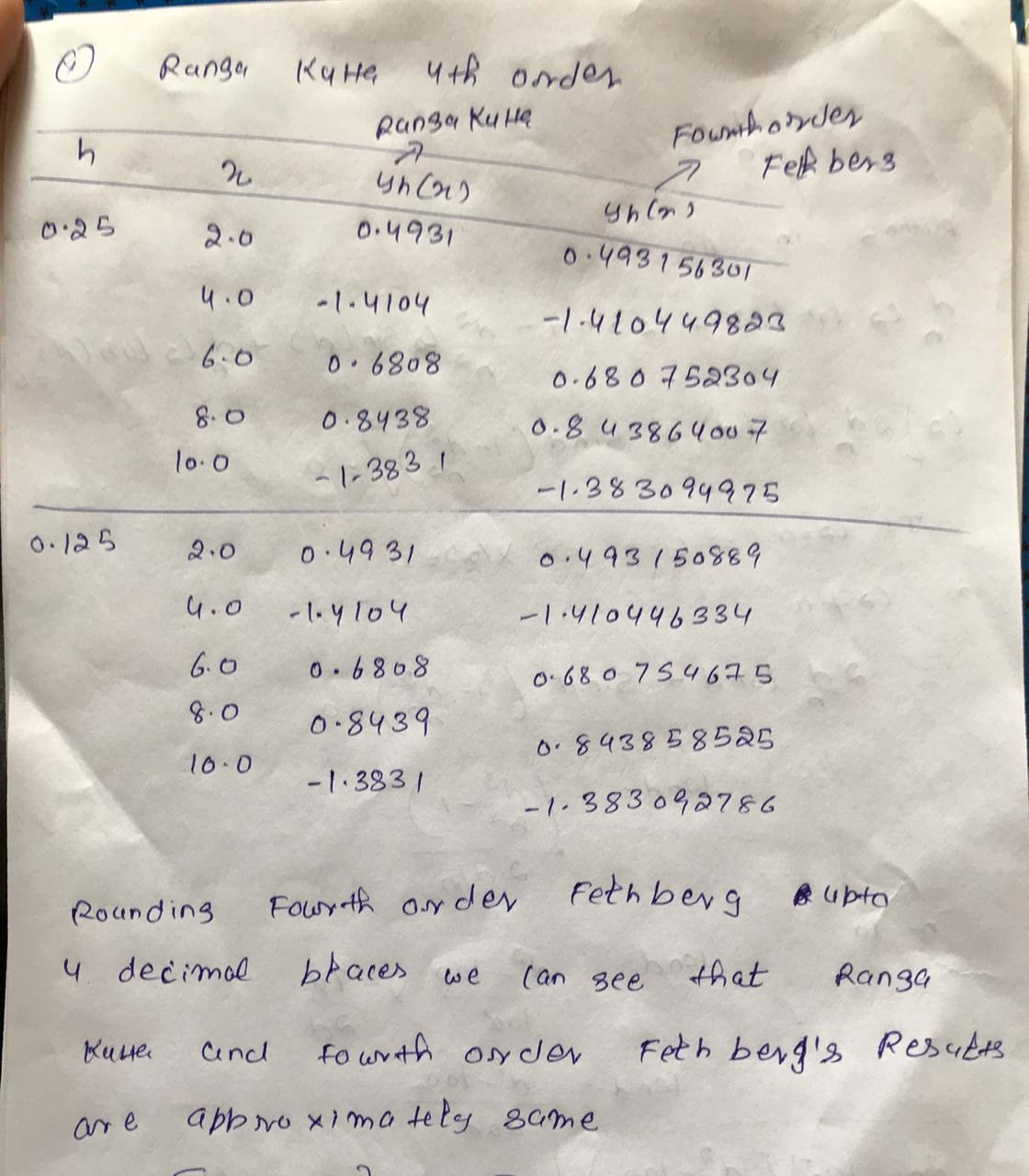##### Add Answer of: Implement the classical procedure, and apply it to equation, Solve it with step sizes bf h...
Similar Homework Help Questions
• ### Write a MATLAB Code to 8.5 (9) LAB. Implement the classical procedure (8.82), and apply it...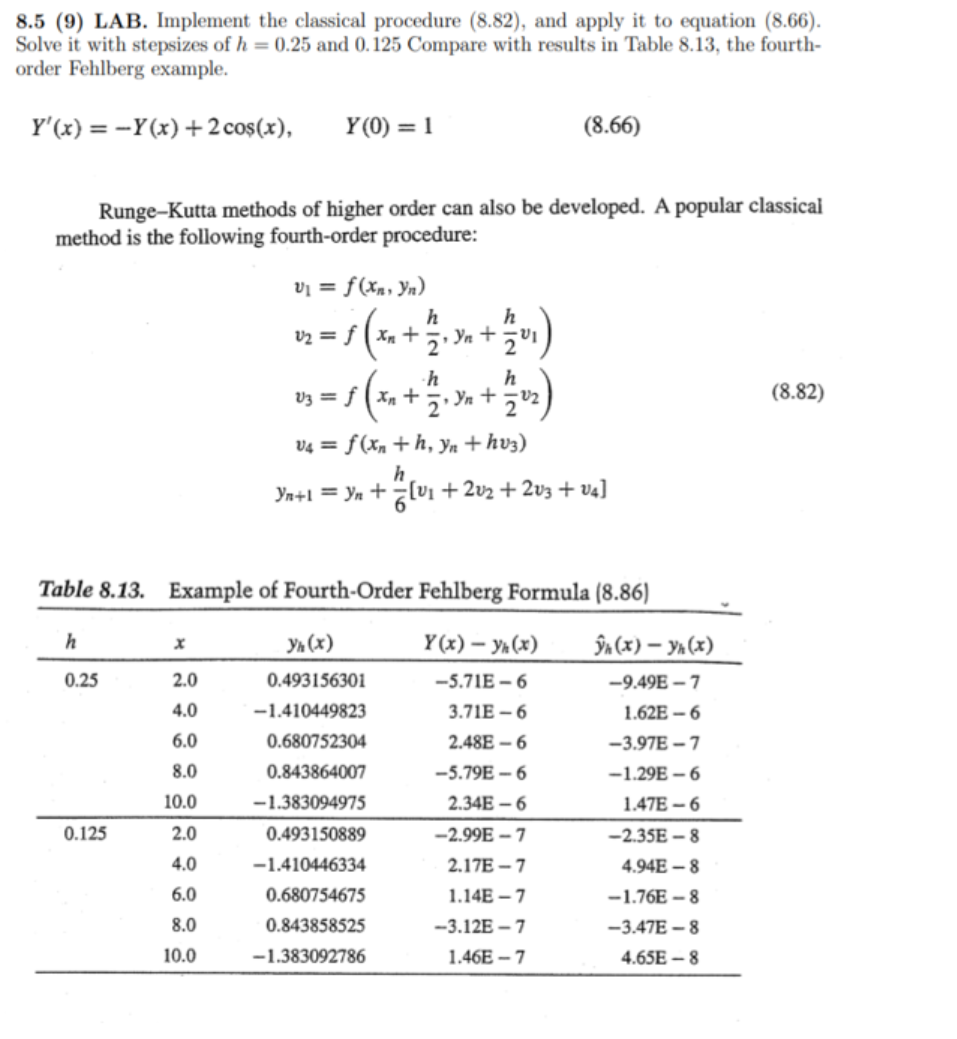Write a MATLAB Code to 8.5 (9) LAB. Implement the classical procedure (8.82), and apply it to equation (8.66). Solve it with stepsizes of h = 0.25 and 0.125 Compare with results in Table 8.13, the fourth- order Fehlberg example. Y'(x) = -Y(x) + 2 co\$(x), Y(0) = 1 (8.66) Runge-Kutta methods of higher order can also be developed. A popular classical method is the following fourth-order procedure: Vi = f (xn, yn) h h v2 = f ( xx...

• ### LAB. Implement the classical procedure (8.82), and apply it to equation (8.66). Solve it with stepsizes...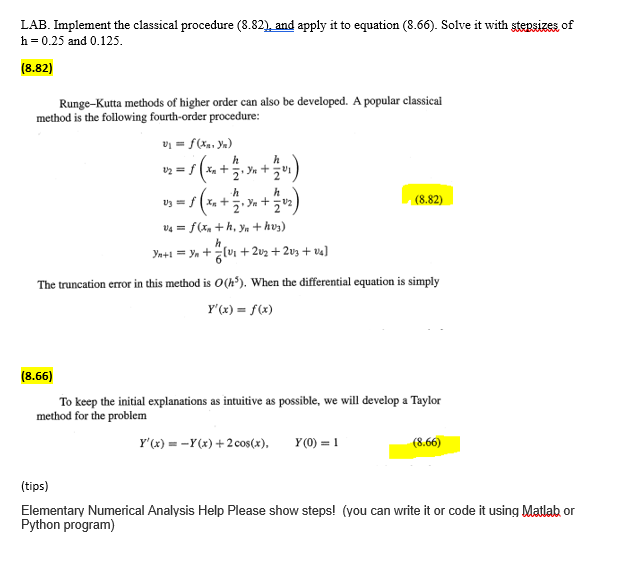LAB. Implement the classical procedure (8.82), and apply it to equation (8.66). Solve it with stepsizes of h = 0.25 and 0.125. (8.82) h h h Runge-Kutta methods of higher order can also be developed. A popular classical method is the following fourth-order procedure: 01 -f(x,y) V2 = 1 (**+ 2 Yu + 2" h lg = f(x+ Yu + (8.82) V4 = f(x+h, yn + hvy) h Yn+1 = y +7(+202 +223 +24] The truncation error in this method...

• ### The both files are included. Where are these which are colorful. Point.h and point.cpp Hor this assignment you are provided the files for a class called point. Download the h and .cpp files and incl...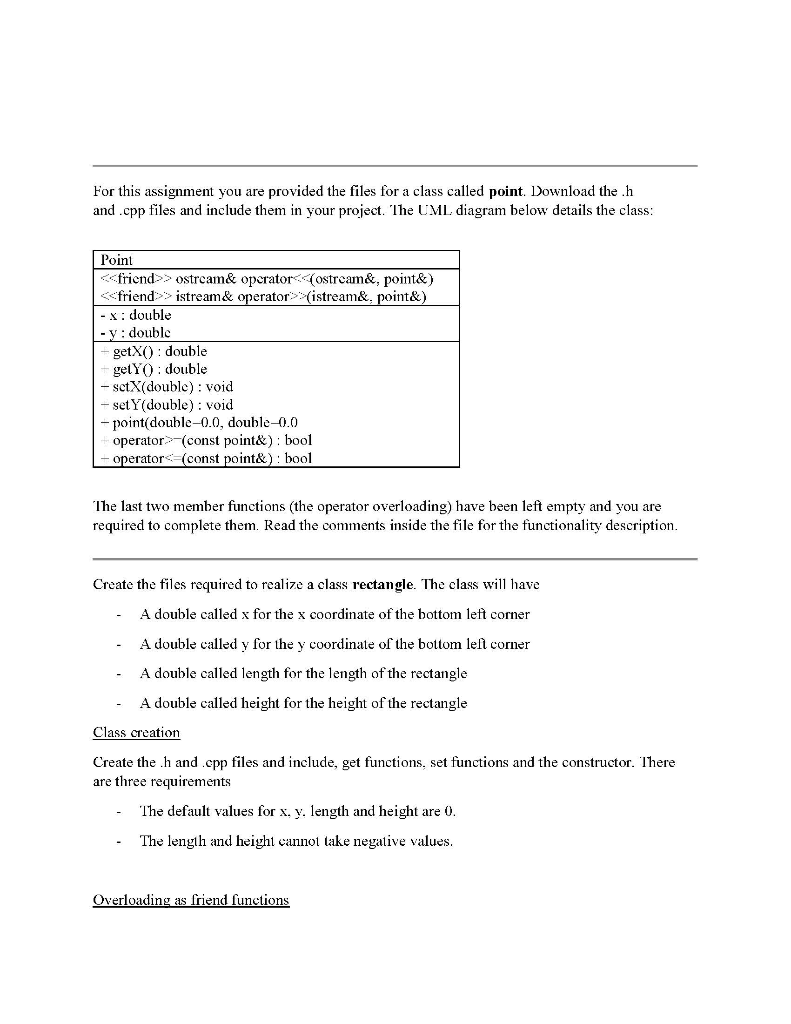The both files are included. Where are these which are colorful. Point.h and point.cpp Hor this assignment you are provided the files for a class called point. Download the h and .cpp files and include them in your project. IheじML diagram below details the class Point くくfriend>> ostream& operator.((ostream&, point&) <ごfriend::. İstream& operator:..イ1stream&-point& - : double - v doublc getX) double getYO double - sctX( double): void - set Y(double) : void - point(double-0.0, double-0.0 operator-(const point& bool perator< const...

• ### can someone help me answer these 5 questions and figire this graph out please? Acid-Base Titration...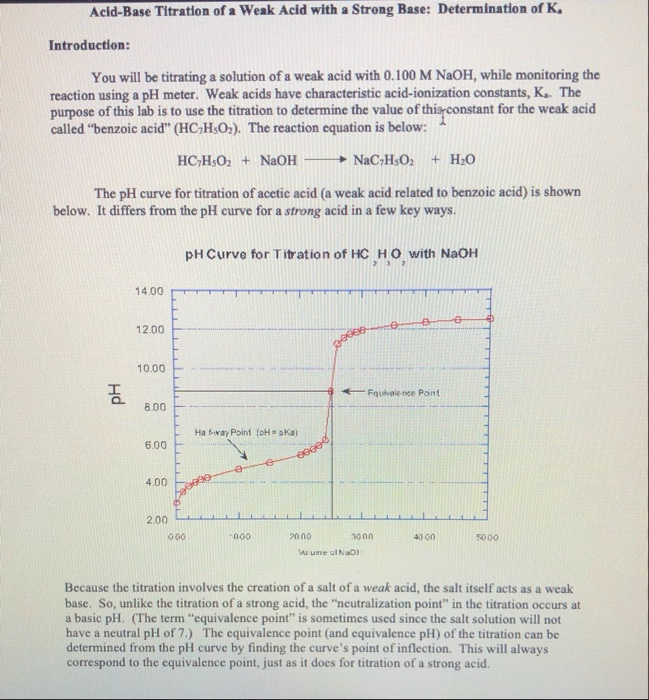can someone help me answer these 5 questions and figire this graph out please? Acid-Base Titration of a Weak Acid with a Strong Base: Determination of K. Introduction: You will be titrating a solution of a weak acid with 0.100 M NaOH, while monitoring the reaction using a pH meter. Weak acids have characteristic acid-ionization constants, K. The purpose of this lab is to use the titration to determine the value of this constant for the weak acid called “benzoic...

• ### I added everything thing. this is the lab question you need to solve. First assigned buffer...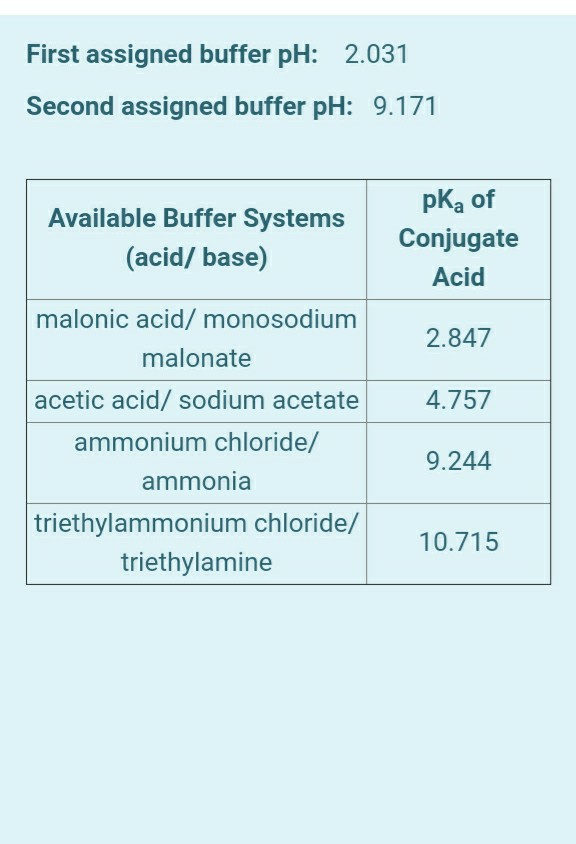I added everything thing. this is the lab question you need to solve. First assigned buffer pH: 2.031 Second assigned buffer pH: 9.171 Available Buffer Systems (acid/ base) pka of Conjugate Acid 2.847 4.757 malonic acid/ monosodium malonate acetic acid/ sodium acetate ammonium chloride/ ammonia triethylammonium chloride/ triethylamine 9.244 10.715 1) Buffer system details: Given pH Name and volume conjugate acid Name and volume conjugate base 2) Calculations for preparation of high capacity buffer system. Introduction In this experiment, you...can someone please help me figure out these 5 questions with the calculations shown please. and i need help with the graph ?? Acid-Base Titration of a Weak Acid with a Strong Base: Determination of K. Introduction: You will be titrating a solution of a weak acid with 0.100 M NaOH, while monitoring the reaction using a pH meter. Weak acids have characteristic acid-ionization constants, K. The purpose of this lab is to use the titration to determine the value...

• ### Principles of Inorganic Chemistry! Week 5 Effect of Temperature on Solubility of a Salt In this experiment, you w...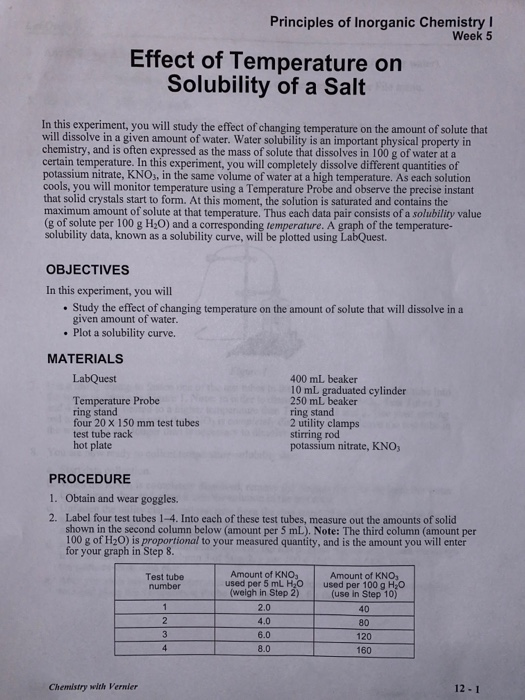Principles of Inorganic Chemistry! Week 5 Effect of Temperature on Solubility of a Salt In this experiment, you will study the effect of changing temperature on the amount of solute that will dissolve in a given amount of water. Water solubility is an important physical property in chemistry, and is often expressed as the mass of solute that dissolves in 100 g of water at a certain temperature. In this experiment, you will completely dissolve different quantities of potassium nitrate,...

Free Homework App